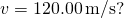16 Waves

16.3 Wave Speed on a Stretched String

Learning Objectives

By the end of this section, you will be able to:

• Determine the factors that affect the speed of a wave on a string
• Write a mathematical expression for the speed of a wave on a string and generalize these concepts for other media

The speed of a wave depends on the characteristics of the medium. For example, in the case of a guitar, the strings vibrate to produce the sound. The speed of the waves on the strings, and the wavelength, determine the frequency of the sound produced. The strings on a guitar have different thickness but may be made of similar material. They have different linear densities, where the linear density is defined as the mass per length,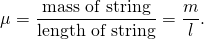In this chapter, we consider only string with a constant linear density. If the linear density is constant, then the mass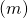of a small length of string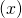is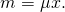For example, if the string has a length of 2.00 m and a mass of 0.06 kg, then the linear density is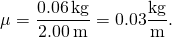If a 1.00-mm section is cut from the string, the mass of the 1.00-mm length is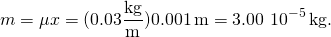The guitar also has a method to change the tension of the strings. The tension of the strings is adjusted by turning spindles, called the tuning pegs, around which the strings are wrapped. For the guitar, the linear density of the string and the tension in the string determine the speed of the waves in the string and the frequency of the sound produced is proportional to the wave speed.

Wave Speed on a String under Tension

To see how the speed of a wave on a string depends on the tension and the linear density, consider a pulse sent down a taut string ((Figure)). When the taut string is at rest at the equilibrium position, the tension in the string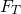is constant. Consider a small element of the string with a mass equal toThe mass element is at rest and in equilibrium and the force of tension of either side of the mass element is equal and opposite.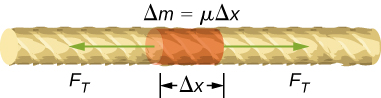Figure 16.13 Mass element of a string kept taut with a tension     . The mass element is in static equilibrium, and the force of tension acting on either side of the mass element is equal in magnitude and opposite in direction.

If you pluck a string under tension, a transverse wave moves in the positive x-direction, as shown in (Figure). The mass element is small but is enlarged in the figure to make it visible. The small mass element oscillates perpendicular to the wave motion as a result of the restoring force provided by the string and does not move in the x-direction. The tensionin the string, which acts in the positive and negative x-direction, is approximately constant and is independent of position and time.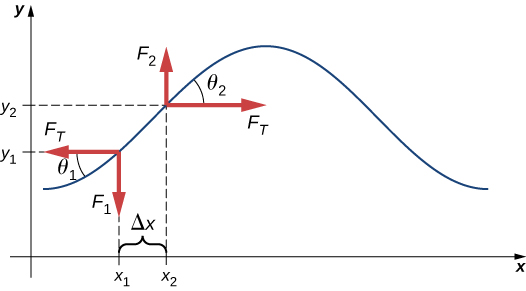Figure 16.14 A string under tension is plucked, causing a pulse to move along the string in the positive x-direction.

Assume that the inclination of the displaced string with respect to the horizontal axis is small. The net force on the element of the string, acting parallel to the string, is the sum of the tension in the string and the restoring force. The x-components of the force of tension cancel, so the net force is equal to the sum of the y-components of the force. The magnitude of the x-component of the force is equal to the horizontal force of tension of the stringas shown in (Figure). To obtain the y-components of the force, note that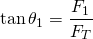and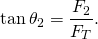The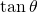is equal to the slope of a function at a point, which is equal to the partial derivative of y with respect to x at that point. Therefore,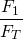is equal to the negative slope of the string at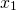and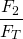is equal to the slope of the string at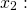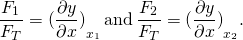The net force is on the small mass element can be written as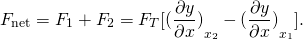Using Newton’s second law, the net force is equal to the mass times the acceleration. The linear density of the string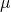is the mass per length of the string, and the mass of the portion of the string is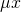,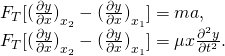Dividing by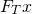and taking the limit as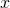approaches zero,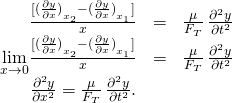Recall that the linear wave equation is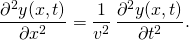Therefore,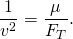Solving for v, we see that the speed of the wave on a string depends on the tension and the linear density.

Speed of a Wave on a String Under Tension

The speed of a pulse or wave on a string under tension can be found with the equation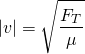whereis the tension in the string andis the mass per length of the string.

Example

The Wave Speed of a Guitar Spring

On a six-string guitar, the high E string has a linear density of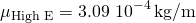and the low E string has a linear density of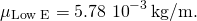(a) If the high E string is plucked, producing a wave in the string, what is the speed of the wave if the tension of the string is 56.40 N? (b) The linear density of the low E string is approximately 20 times greater than that of the high E string. For waves to travel through the low E string at the same wave speed as the high E, would the tension need to be larger or smaller than the high E string? What would be the approximate tension? (c) Calculate the tension of the low E string needed for the same wave speed.

Strategy

1. The speed of the wave can be found from the linear density and the tension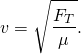2. From the equation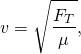if the linear density is increased by a factor of almost 20, the tension would need to be increased by a factor of 20.

3. Knowing the velocity and the linear density, the velocity equation can be solved for the force of tension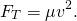Solution

1. Use the velocity equation to find the speed: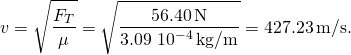2. The tension would need to be increased by a factor of approximately 20. The tension would be slightly less than 1128 N.
3. Use the velocity equation to find the actual tension: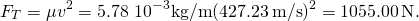This solution is within

*** QuickLaTeX cannot compile formula:
$7\text{%}$

*** Error message:
File ended while scanning use of \text@.
Emergency stop.

of the approximation.

Significance

The standard notes of the six string (high E, B, G, D, A, low E) are tuned to vibrate at the fundamental frequencies (329.63 Hz, 246.94Hz, 196.00Hz, 146.83Hz, 110.00Hz, and 82.41Hz) when plucked. The frequencies depend on the speed of the waves on the string and the wavelength of the waves. The six strings have different linear densities and are “tuned” by changing the tensions in the strings. We will see in Interference of Waves that the wavelength depends on the length of the strings and the boundary conditions. To play notes other than the fundamental notes, the lengths of the strings are changed by pressing down on the strings.

The wave speed of a wave on a string depends on the tension and the linear mass density. If the tension is doubled, what happens to the speed of the waves on the string?

Since the speed of a wave on a taunt string is proportional to the square root of the tension divided by the linear density, the wave speed would increase by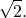Speed of Compression Waves in a Fluid

The speed of a wave on a string depends on the square root of the tension divided by the mass per length, the linear density. In general, the speed of a wave through a medium depends on the elastic property of the medium and the inertial property of the medium.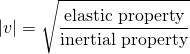The elastic property describes the tendency of the particles of the medium to return to their initial position when perturbed. The inertial property describes the tendency of the particle to resist changes in velocity.

The speed of a longitudinal wave through a liquid or gas depends on the density of the fluid and the bulk modulus of the fluid,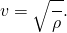Here the bulk modulus is defined as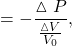where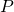is the change in the pressure and the denominator is the ratio of the change in volume to the initial volume, and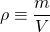is the mass per unit volume. For example, sound is a mechanical wave that travels through a fluid or a solid. The speed of sound in air with an atmospheric pressure of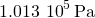and a temperature of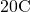is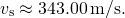Because the density depends on temperature, the speed of sound in air depends on the temperature of the air. This will be discussed in detail in Sound.

Summary

• The speed of a wave on a string depends on the linear density of the string and the tension in the string. The linear density is mass per unit length of the string.
• In general, the speed of a wave depends on the square root of the ratio of the elastic property to the inertial property of the medium.
• The speed of a wave through a fluid is equal to the square root of the ratio of the bulk modulus of the fluid to the density of the fluid.
• The speed of sound through air at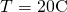is approximately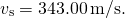Conceptual Questions

If the tension in a string were increased by a factor of four, by what factor would the wave speed of a wave on the string increase?

[hidden-answer a=”936911″]The wave speed is proportional to the square root of the tension, so the speed is doubled.[/hidden-answer]

Does a sound wave move faster in seawater or fresh water, if both the sea water and fresh water are at the same temperature and the sound wave moves near the surface?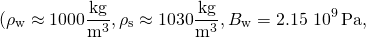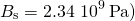Guitars have strings of different linear mass density. If the lowest density string and the highest density string are under the same tension, which string would support waves with the higher wave speed?

[hidden-answer a=”177839″]Since the speed of a wave on a string is inversely proportional to the square root of the linear mass density, the speed would be higher in the low linear mass density of the string.[/hidden-answer]

Shown below are three waves that were sent down a string at different times. The tension in the string remains constant. (a) Rank the waves from the smallest wavelength to the largest wavelength. (b) Rank the waves from the lowest frequency to the highest frequency.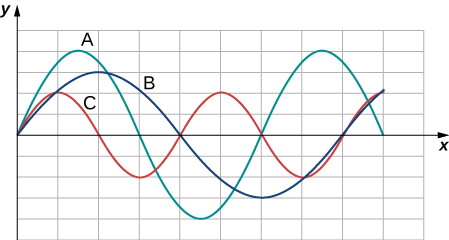Electrical power lines connected by two utility poles are sometimes heard to hum when driven into oscillation by the wind. The speed of the waves on the power lines depend on the tension. What provides the tension in the power lines?

[hidden-answer a=”960058″]The tension in the wire is due to the weight of the electrical power cable.[/hidden-answer]

Two strings, one with a low mass density and one with a high linear density are spliced together. The higher density end is tied to a lab post and a student holds the free end of the low-mass density string. The student gives the string a flip and sends a pulse down the strings. If the tension is the same in both strings, does the pulse travel at the same wave velocity in both strings? If not, where does it travel faster, in the low density string or the high density string?

Problems

Transverse waves are sent along a 5.00-m-long string with a speed of 30.00 m/s. The string is under a tension of 10.00 N. What is the mass of the string?

A copper wire has a density of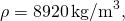a radius of 1.20 mm, and a length L. The wire is held under a tension of 10.00 N. Transverse waves are sent down the wire. (a) What is the linear mass density of the wire? (b) What is the speed of the waves through the wire?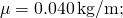b.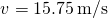A piano wire has a linear mass density of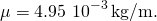Under what tension must the string be kept to produce waves with a wave speed of 500.00 m/s?

A string with a linear mass density of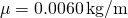is tied to the ceiling. A 20-kg mass is tied to the free end of the string. The string is plucked, sending a pulse down the string. Estimate the speed of the pulse as it moves down the string.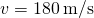A cord has a linear mass density of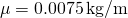and a length of three meters. The cord is plucked and it takes 0.20 s for the pulse to reach the end of the string. What is the tension of the string?

A string is 3.00 m long with a mass of 5.00 g. The string is held taut with a tension of 500.00 N applied to the string. A pulse is sent down the string. How long does it take the pulse to travel the 3.00 m of the string?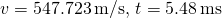Two strings are attached to poles, however the first string is twice as long as the second. If both strings have the same tension and mu, what is the ratio of the speed of the pulse of the wave from the first string to the second string?

Two strings are attached to poles, however the first string is twice the linear mass density mu of the second. If both strings have the same tension, what is the ratio of the speed of the pulse of the wave from the first string to the second string?

0.707

Transverse waves travel through a string where the tension equals 7.00 N with a speed of 20.00 m/s. What tension would be required for a wave speed of 25.00 m/s?

Two strings are attached between two poles separated by a distance of 2.00 m as shown below, both under the same tension of 600.00 N. String 1 has a linear density of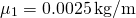and string 2 has a linear mass density of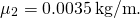Transverse wave pulses are generated simultaneously at opposite ends of the strings. How much time passes before the pulses pass one another?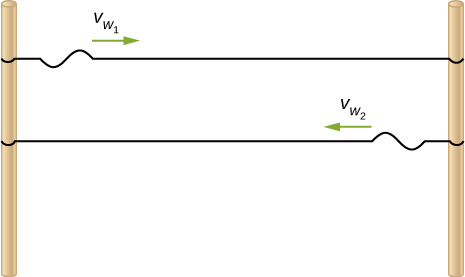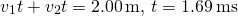Two strings are attached between two poles separated by a distance of 2.00 meters as shown in the preceding figure, both strings have a linear density of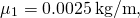the tension in string 1 is 600.00 N and the tension in string 2 is 700.00 N. Transverse wave pulses are generated simultaneously at opposite ends of the strings. How much time passes before the pulses pass one another?

The note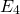is played on a piano and has a frequency of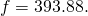If the linear mass density of this string of the piano is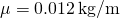and the string is under a tension of 1000.00 N, what is the speed of the wave on the string and the wavelength of the wave?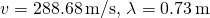Two transverse waves travel through a taut string. The speed of each wave is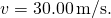A plot of the vertical position as a function of the horizontal position is shown below for the time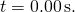(a) What is the wavelength of each wave? (b) What is the frequency of each wave? (c) What is the maximum vertical speed of each string?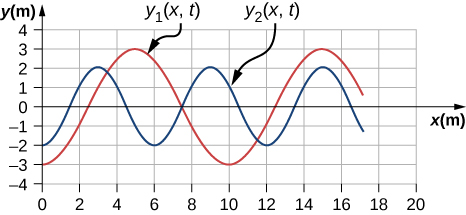A sinusoidal wave travels down a taut, horizontal string with a linear mass density of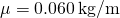. The maximum vertical speed of the wave is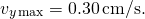The wave is modeled with the wave equation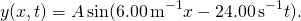(a) What is the amplitude of the wave? (b) What is the tension in the string?

a.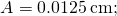b.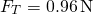The speed of a transverse wave on a string is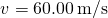and the tension in the string is. What must the tension be to increase the speed of the wave to